## Spherical Bessel Differential Equation

Take the Helmholtz Differential Equation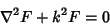(1)

in Spherical Coordinates. This is just Laplace's Equation in Spherical Coordinates with an additional term,(2)

Multiply through by,(3)

This equation is separable in. Call the separation constant,(4)

Now multiply through by,(5)

This is the Spherical Bessel Differential Equation. It can be transformed by letting, then(6)

Similarly,(7)

so the equation becomes(8)

Now look for a solution of the form, denoting a derivative with respect toby a prime,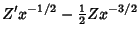(9)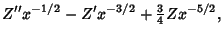(10)

so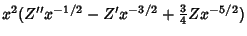(11)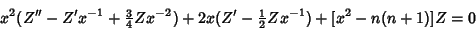(12)(13)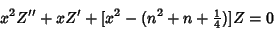(14)(15)

But the solutions to this equation are Bessel Functions of half integral order, so the normalized solutions to the original equation are(16)

which are known as Spherical Bessel Functions. The two types of solutions are denoted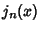(Spherical Bessel Function of the First Kind) or(Spherical Bessel Function of the Second Kind), and the general solution is written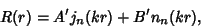(17)

where(18)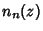(19)

See also Spherical Bessel Function, Spherical Bessel Function of the First Kind, Spherical Bessel Function of the Second Kind

References

Abramowitz, M. and Stegun, C. A. (Eds.). Handbook of Mathematical Functions with Formulas, Graphs, and Mathematical Tables, 9th printing. New York: Dover, p. 437, 1972.